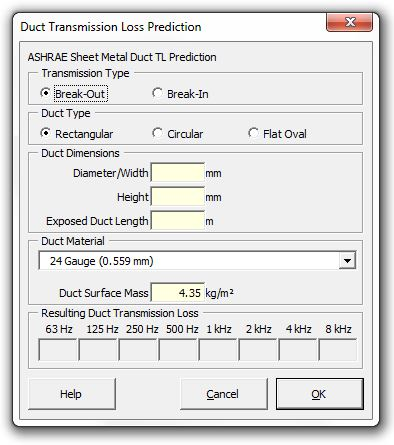### Strutt Help

Duct Transmission Loss Prediction    1/1

Strutt|Mechanical Services|Duct Breakout|Duct Transmission Loss inserts duct transmission loss values into the active row of the worksheet.Duct transmission loss can be calculated for Break-Out and Break-In.
Both calculations require the following inputs:

• Duct type
• Dimensions (mm)
• Exposed duct length (m)
• Material (Optional)
• Duct surface mass (kg/m3)

The maximum allowable value for TLout is 50 dB. If the value exceeds 50 dB, TL is set equal to 50 dB.

Rectangular Ducts

Break-out TL is divided into two regions, a region where plane mode transmission within the duct is dominant and a region where mutli-mode transmission is dominant. The frenquency fL that divides these two regions is given by:

f_L = 24134/sqrt(ab)

where:
a is the major dimension (larger duct cross-sectional dimension), inch
b is the minor dimension (smaller duct cross-sectional dimension), inch

If f < f_L, the plane mode predominates and TL_(out) is given by:

TL_(out) = 10log_10((q^2 f)/(a + b)) + 17

where:
q is the mass/unit area (lb/ft2) of the duct walls

If f > f_L, multi-mode transmission predominates and TL_(out) is calculated by:

TL_(out) = 20log_10(q f) - 31

where q and f are as specified above.

The minimum value of TLout occurs when the incident sound power on the duct from the surrounding space equals the sound power that travels along the duct both upstream and downstream, from the point where the sound enters the duct. This is calculated by:

TL_(out,min) = 10log_10[24 L (1/a + 1/b)]

Break-in TL is divided into two regions, separated by a cutoff frequency, f1, which is given by:
f_1 = 6764/a

If f < f_1:
TL_(i n) = larg er \ of {(TL_(out) - 4 - 10log_10(a/b) + 20log_10(f/f_1), \ ),(10log_10[12L(1/a + 1/b)], \ ):}

Where,
L is the exposed length of duct, ft

If f > f_1:

TL_(i n) = TL_(out) - 3

Circular Ducts:

Break-out TL is calculated by:

TL_(out) = "larger of" {(17.6log_10 q - 49.8log_10 f - 55.3log_10 d + C_0
, \ ),(17.6log_10 q - 6.6log_10 f - 36.9log_10 d + 97.4, \ ):}

where,
q and f are as defined for rectangular ducts
d is the inside duct diameter
C_0 is 231.65

When the diameter of the duct is greater than 26 inches and the frequency is 4000 Hz, TLout is given by:

TL_(out) = 17.6log_10 q - 36.9log_10 d + 90.6

Break-in TL is divided into two regions, separated by a cutoff frequency f1, which is given by:

f_1 = 7929/d

If f < f_1:

TL_(i n) = larg er \ of{(TL_(out) - 4 + 20log_10(f/f_1), \ ),(10log_10((2L)/d), \ ):}

If f > f_1:

TL_(i n) = TL_(out) - 3

Flat Oval Ducts:

The minimum break-out TL is calculated by:

TL_(out,min) = 10log_10(A_0/A_i)

where:
A_0 = 12L[2(a - b) + pi b]

A_i = b(a - b) + (pi b^2)/4

The low to mid frequency TL is calculated by:

TL_(out) = 10log_10((q^2 f)/(sigma^2 P)) + 20

where,

P = 2(a - b) + pi b

sigma = 1/(1 + ((pi b)/(2(a - b))))

The upper frequency limit of applicability is:

f_L = 8115/b

Break-in TL is divided into two regions, separated by a cutoff frequency f1, which is given by:

f_1 = 6764/((a - b)sqrt(1 + ((pi b)/(2(a - b))))), \ fo r \ a/b >= 2

When f < f_1,

TL_(i n) = larg er \ of {(TL_(out) - 4 + 10 log_10(f^2 A_i) - 81, \ ),(10log_10((6PL)/A_i), \ ):}

When f > f_1,

TL_(i n) = TL_(out) - 3

References:

• ASHRAE Algorithms for HVAC Acoustics (1991)